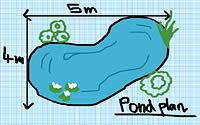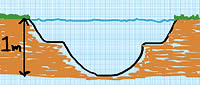# Pond Calculator

Sketch out a plan of how you want the pond to look and calculate the width and length of your pond.

## Pond Liner Calculator

#### Got Imperial Measurements?

If your measurements are in feet and inches, converting it is easy.
1 foot = 0.3 metres, so just multiply your measurement in feet by 0.3 to get the value in metres. For example 2 feet = 2 × 0.3 = 0.6 metres.

### Results

Pond volume (in litres)
Pond liner length (in metres)
Pond liner width (in metres)
How to calculate the pond liner size
Learn the easy way to calculate it.

The accompanying diagram shows a pond with a maximum length of 5 metres and a maximum width of 4 metres. The final measurement you will need is the maximum depth of your pond, the diagram to the right shows a maximum pond depth of 1 metre.

Liner length = max length + (2 x max depth) + 1 (for overlap)
Liner width = max width + (2 x max depth) + 1 (for overlap)

So for a 5 meter by 4 meter pond it would be:

Pond liner length = 5+2+1 = 8 metres
Pond liner width = 4+2+1 = 7 metres

You'd need a pond liner that is 8 metres x 7 metresNeed some help?
From building your own pond to calculating what you need, we can help.#### Why getting a pond is a great investment

Another line here briefly explaining the guides.#### Building a pond is easy with our simple guide#### Why do I need a pond liner?

LDPE, PVC, Rubber? Learn about the benefits and characteristics of different type of pond liner.